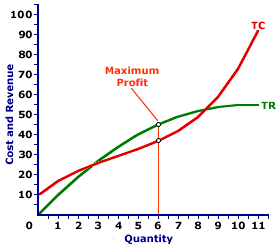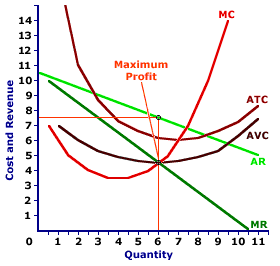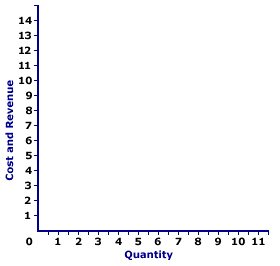Wednesday  September 27, 2023
 AmosWEB means Economics with a Touch of Whimsy!INDUSTRY: A group of firms producing goods or services that are close substitutes-in-consumption. The similarity of the products makes it possible to analyze the production in a market framework. An industry can be broadly defined, such as the manufacturing industry, or narrowly specified, such as the root beer industry. For most economic analysis the term industry is used interchangeably with the term market.MONOPOLY, PROFIT MAXIMIZATION:

A monopoly is presumed to produce the quantity of output that maximizes economic profit--the difference between total revenue and total cost. This production decision can be analyzed directly with economic profit, by identifying the greatest difference between total revenue and total cost, or by the equality between marginal revenue and marginal cost.
Profit Maximization
Profit CurveTotal CurvesMarginal CurvesThe profit-maximizing level of output is a production level that achieves the greatest level of economic profit given existing market conditions and production cost. For a monopoly, this entails adjusting the price and corresponding production level to achieved the desired match between total revenue and total cost.

### Three Views

Profit-maximizing output can be identified in one of three ways--directly with economic profit, with a comparison of total revenue and total cost, and with comparison of marginal revenue and marginal cost.

This exhibit illustrates how it can be identified for a monopoly, such as that operated by Feet-First Pharmaceutical, a well-known monopoly supplier of Amblathan-Plus, the only cure for the deadly (but hypothetical) foot ailment known as amblathanitis. Feet-First Pharmaceutical is the exclusive producer of Amblathan-Plus, meaning that it is a price maker and the demand curve it faces is the market demand curve.

The top panel presents the profit curve. The middle panel presents total revenue and total cost curves. The bottom panel presents average revenue and average total cost curves. In all three panels, Feet-First Pharmaceutical maximizes when producing 6 ounces of Amblathan-Plus.

• Profit: First, profit maximization can be illustrated with a direct evaluation of profit. If the profit curve is at its peak, then profit is maximized. In the top panel, the profit curve achieves its highest level at 6 ounces of Amblathan-Plus. At other output levels, profit is less.

• Total Revenue and Total Cost: Second, profit maximization can be identified by a comparison of total revenue and total cost. The quantity of output that achieves the greatest difference of total revenue over total cost is profit maximization. In the middle panel, the vertical gap between the total revenue and total cost curves is the greatest at 6 ounces of Amblathan-Plus. For smaller or larger output levels, the gap is either less or the total cost curve lies above the total revenue curve.

• Marginal Revenue and Marginal Cost: Third, profit maximization can be identified by a comparison of marginal revenue and marginal cost. If marginal revenue is equal to marginal cost, then profit cannot be increased by changing the level of production. Increasing production adds more to cost than revenue, meaning profit declines. Decreasing production subtracts more from revenue than from cost, meaning profit also declines. In the bottom panel, the marginal revenue and marginal cost curves intersect at 6 ounces of Amblathan-Plus. At larger or smaller output levels, marginal cost exceeds marginal revenue or marginal revenue exceeds marginal cost.

### More on the Marginal View

Further analysis of the marginal approach to analyzing profit maximization provides further insight into the short-run production decision of a monopoly.

First, consider the logic behind using marginals to identify profit maximization.

1. Marginal revenue indicates how much total revenue changes by producing one more or one less unit of output.

2. Marginal cost indicates how much total cost changes by producing one more or one less unit of output.

3. Profit increases if marginal revenue is greater than marginal cost and profit decreases if marginal revenue is less than marginal cost.

4. Profit neither increases nor decreases if marginal revenue is equal to marginal cost.

5. As such, the production level that equates marginal revenue and marginal cost is profit maximization.
Profit Maximization,
The Marginal ViewWith this in mind, now consider this exhibit to the right, which will eventually contain the marginal revenue and marginal cost curves for Feet-First Pharmaceutical's Amblathan-Plus production.
• Average Revenue: First up is the average revenue curve, which can be seen with a click of the [Average Revenue] button. Because Feet-First Pharmaceutical is a monopoly, this average revenue curve is the market demand curve for Amblathan-Plus, which is negatively-sloped due to the law of demand.

• Marginal Revenue: A click of the [Marginal Revenue] button reveals the green line labeled MR that depicts the marginal revenue Feet-First Pharmaceutical receives from Amblathan-Plus production. Because Feet-First Pharmaceutical is a price maker, this marginal revenue curve is also a negatively-sloped line, and it lies beneath the average revenue (market demand) curve.

• Marginal Cost: The marginal cost curve is U-shaped, reflecting the principles of short-run production. Click the [Marginal Cost] button to add this curve to the diagram. It has a negative slope for small amounts of output, then the slope is positive for larger quantities due to the law of diminishing marginal returns.

• Profit Maximization: Profit is maximized at the quantity of output found at the intersection of the marginal revenue and marginal cost curves, which is 6 ounces of Amblathan-Plus. Click the [Profit Max] button to highlight this production level. This is the same profit-maximizing level identified using the total revenue and total cost curves and the profit curve.

Consider what results if marginal revenue is not equal to marginal cost:

• If marginal revenue is greater than marginal cost, as is the case for small quantities of output, then the firm can increase profit by increasing production. Extra production adds more to revenue than to cost, so profit increases.

• If marginal revenue is less than marginal cost, as is the case for large quantities of output, then the firm can increase profit by decreasing production. Reducing production reduces revenue less than it reduces cost, so profit increases.

• If marginal revenue is equal to marginal cost, then the firm cannot increase profit by producing more or less output. Profit is maximized.

 <= MONOPOLY, PROFIT ANALYSIS MONOPOLY, REALISM =>Recommended Citation:

MONOPOLY, PROFIT MAXIMIZATION, AmosWEB Encyclonomic WEB*pedia, http://www.AmosWEB.com, AmosWEB LLC, 2000-2023. [Accessed: September 27, 2023].

Check Out These Related Terms...

Or For A Little Background...

And For Further Study...
Search Again?YELLOW CHIPPEROON[What's This?] Today, you are likely to spend a great deal of time looking for a downtown retail store hoping to buy either a three-hole paper punch or decorative picture frames. Be on the lookout for vindictive digital clocks with revenge on their minds.Your Complete ScopeGeneral Electric is the only stock from the original 1896 Dow Jones Industrial Average remaining in the current index."Don't judge each day by the harvest you reap but by the seeds that you plant. "-- Robert Louis Stephenson, writerBOABasic Ordering AgreementA PEDestrian's Guide Xtra CreditTell us what you think about AmosWEB. Like what you see? Have suggestions for improvements? Let us know. Click the User Feedback link.| | | | | | | | | | |
| | | |

Thanks for visiting AmosWEB